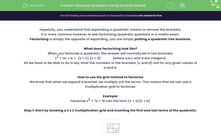# Factorise Quadratics Using the Grid Method

In this worksheet, students will explore how to factorise a quadratic of the form x2 + bx + c by using the grid method.Key stage:  KS 4

Year:  GCSE

GCSE Subjects:   Maths

GCSE Boards:   AQA, Eduqas, OCR, Pearson Edexcel,

Curriculum topic:   Algebra

Curriculum subtopic:   Solving Equations and Inequalities Algebraic Expressions

Popular topics:   Factors worksheets

Difficulty level:#### Worksheet Overview

Hopefully, you understand that expanding a quadratic means to remove the brackets.

It is more common however to see factorising quadratic questions in a maths exam.

Factorising is simply the opposite of expanding, you are simply putting a quadratic into brackets.

What does factorising look like?

When you factorise a quadratic, the answer will normally be in two brackets.

x2 + ax + b =  (x + c) (x + d)             {where a,b,c and d are integers}

All we have to be able to do is say what the numbers in the brackets (c and d) are for any given values of a and b.

How to use the grid method to factorise

We know that when we expand a bracket, we multiply out the terms. This means that we can use a multiplication grid to factorise.

Example:

Factorise x2 + 7x + 10 into the form (x + a)(x + b)

Step 1: Start by drawing a 2 x 2 multiplication grid and inserting the first and last terms of the quadratic.Step 2: Find what must go into the other two cells.

These terms have to add to the middle term in the quadratic (7x)

and multiply to the product of the two numbers we have already put into the grid (10x2).

The only two terms that satisfy this are 5x and 2x. (5x + 2x = 7x and 5x x 2x = 10x2)Step 3: Factorise each row and column.Step 4: Put together the grid.Step 5: Create the brackets from the grid.

The two brackets are given by the terms in red.

So x2 + 7x + 10 ≡ (x + 5)(x + 2)

This could also be written the other way round

x2 + 7x + 10 ≡ (x + 2)(x + 5)

You can quite happily just swap these brackets round (but not the numbers inside them)

This seems very long winded and time consuming!

You will probably have seen quicker methods than this for factorising quadratics, however this method will work for every quadratic factorisation. It works for quadratics with negatives as well as quadratics such as 6x2 + 7x + 2 and even 2x2 + 3xy + 2y2. It’s definitely worth learning!

Let's try some questions.

### What is EdPlace?

We're your National Curriculum aligned online education content provider helping each child succeed in English, maths and science from year 1 to GCSE. With an EdPlace account you’ll be able to track and measure progress, helping each child achieve their best. We build confidence and attainment by personalising each child’s learning at a level that suits them.

Get started#### Popular Maths topics

••••